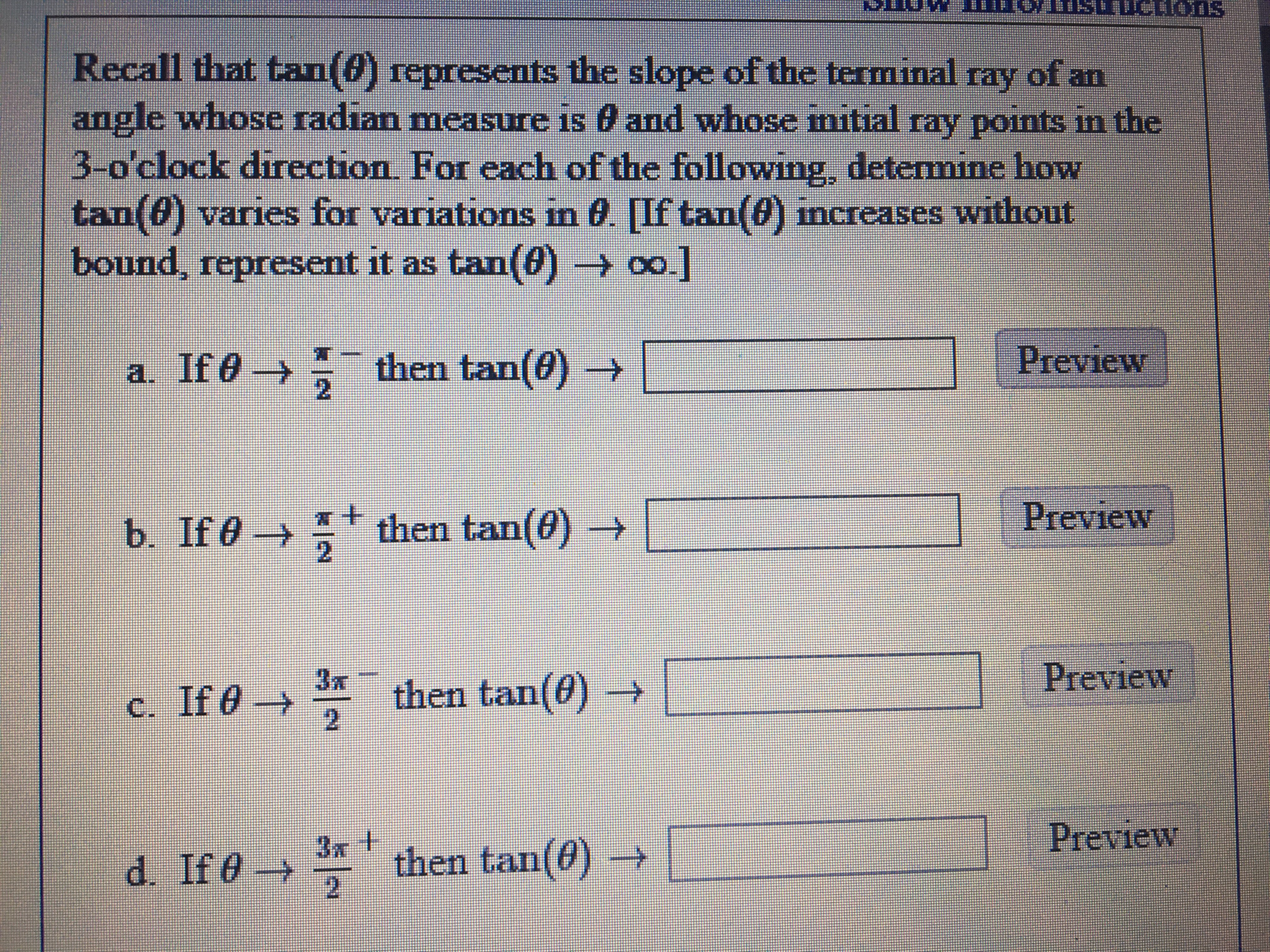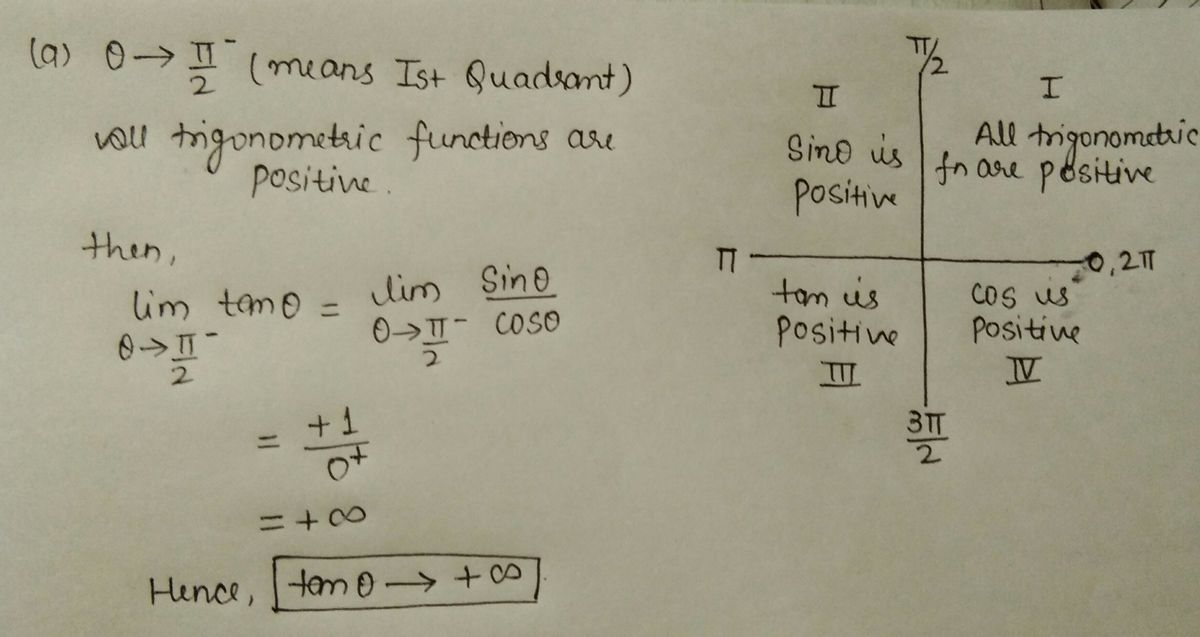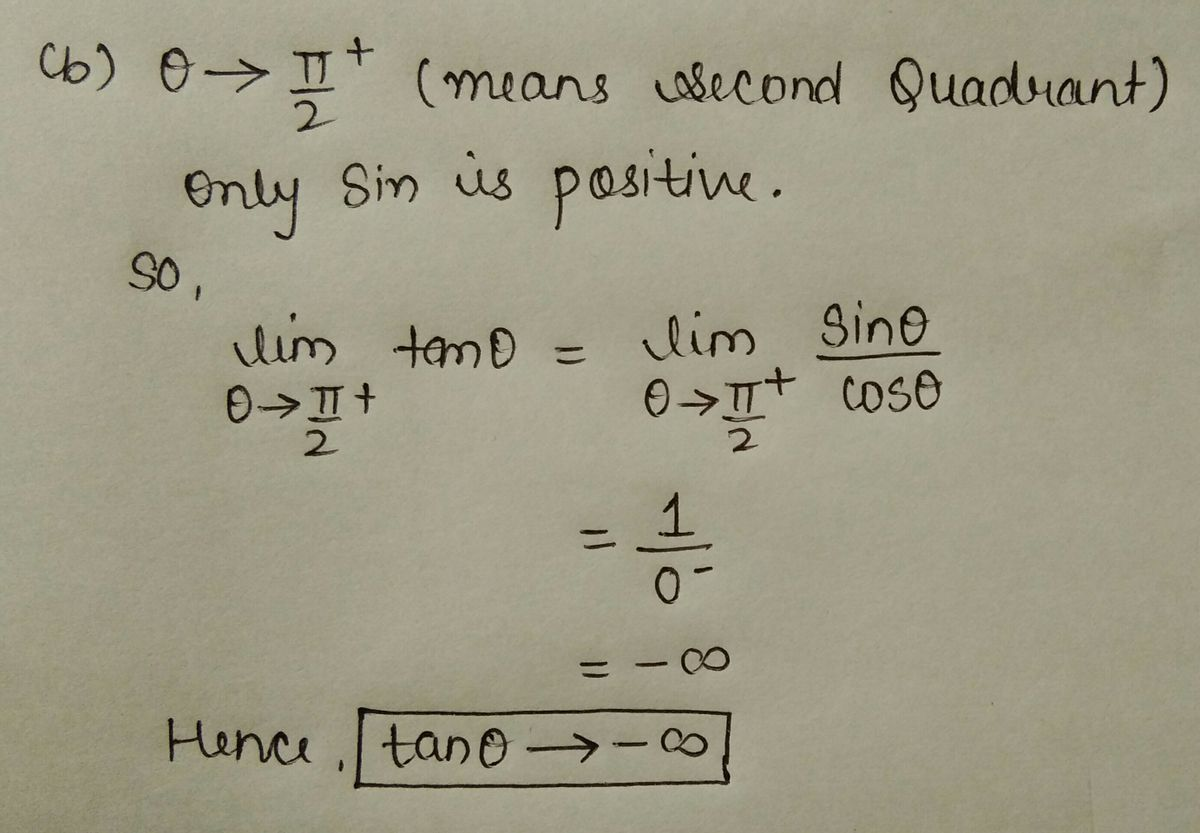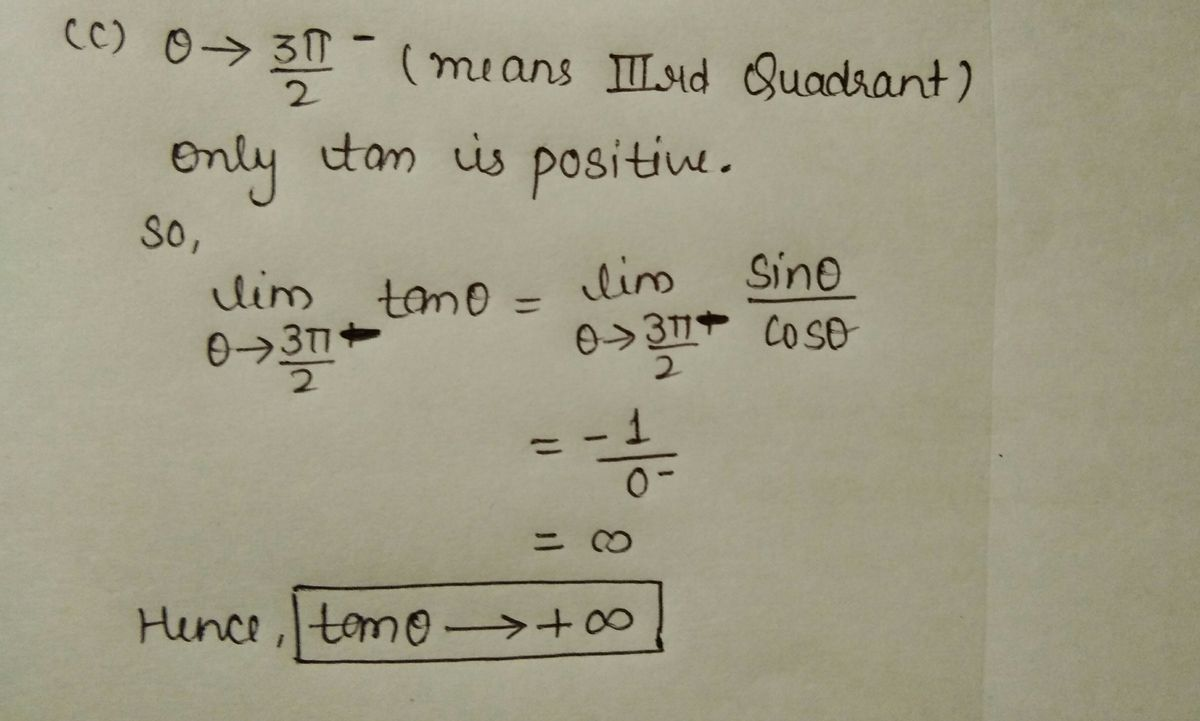# Recall that tan(0) represents the slope of the terminal ray of anangle whose radian measure is 0 and whose initial ray points in the3-o'clock direction For each of the following, determine howtan(0) varies for variations in 0. [If tan(0) mcreases withoutbound, represent it as tan(60)→ 0o.]a. If 0→then tan(0)b. If 0 then tan(0)2.->3지Previewc. If 0 then tan(0)2.Preview3xd. If 0then tan(0) -

Question
1 viewshelp_outlineImage TranscriptioncloseRecall that tan(0) represents the slope of the terminal ray of an angle whose radian measure is 0 and whose initial ray points in the 3-o'clock direction For each of the following, determine how tan(0) varies for variations in 0. [If tan(0) mcreases without bound, represent it as tan(60)→ 0o.] a. If 0→ then tan(0) b. If 0 then tan(0) 2. -> 3지 Preview c. If 0 then tan(0) 2. Preview 3x d. If 0 then tan(0) - fullscreen
check_circle

Step 1Step 2Step 3...

### Want to see the full answer?

See Solution

#### Want to see this answer and more?

Solutions are written by subject experts who are available 24/7. Questions are typically answered within 1 hour.*

See Solution
*Response times may vary by subject and question.
Tagged in